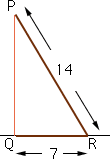Subject: geometry Name: monica I Who is asking: Student Level: Secondary Question: What is the measure of the angle determined by a 14 inch segment and its projection into a plane if the length,in inches, of the projection into the plane is 7 inches? Is there a formula to follow ? Please help? Hi Monica, I assume that the projection is perpendicular to the plane so that triangel PQR below has a right angle at Q.Notice that in this right angled triangle the hypotenuse is twice the length of one of the sides, QR. Thus this triangle is similar to the right angled triangle with one side of length 1 and the hypotenuse of length 2. By the theorem of Pythagoras the length of the third side is the square root of 3. Do you know the angle measures in this triangle? Cheers, Penny Go to Math Central